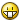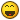# Correcting A Slight Problem## Recommended PostsI am writing a small program for my math teacher to solve quadratic equations...

Here is my code:

```#include "GUIConstants.au3"

\$window1 = GUICreate("Quadratic Solver -Version 1.0", 700, 400)
GUISetBkColor(0x000000)
GUICtrlCreateLabel("Quadratic Solver", 255, 10, 300, 300)
GUICtrlSetColor(-1,0xFFFFFF)
GUICtrlSetFont(-1, 14, 40)
GUICtrlCreateLabel("(Created by Reaper)", 263, 40, 300, 300)
GUICtrlSetColor(-1,0xFFFFFF)
GUICtrlSetFont(-1, 10, 10)
\$instructions = GUICtrlCreateButton("&Instructions", 10, 240, 90, 25)
\$clearbox = GUICtrlCreateButton("&Clear Box", 10, 270, 90, 25)
GUICtrlCreateLabel("Created by Reaper. -Period 2", 160, 340, 400, 400)
GUICtrlSetColor(-1,0xFFFFFF)
GUICtrlSetFont(-1, 10, 40)
\$solve = GUICtrlCreateButton("&Solve", 10, 300, 90, 25)
\$a = GUICtrlCreateInput("", 160, 120, 150, 30); a's value (user imput)
\$b = GUICtrlCreateInput("", 160, 160, 150, 30); b's value (user input)
\$c = GUICtrlCreateInput("", 160, 200, 150, 30); c's value (user input)

\$style = BitOr(\$ES_WANTRETURN, \$WS_VSCROLL, \$ES_AUTOVSCROLL)

\$window2 = GUICreate("Quadratic Solver -Help File", 700, 400)
GUISetBkColor(0x000000)
GUICtrlCreateLabel("Instructions", 285, 10, 300, 300)
GUICtrlSetColor(-1,0xFFFFFF)
GUICtrlSetFont(-1, 14, 10)
\$help = GUICtrlCreateEdit("This program determines the quadratic roots of an equation given the 'a', 'b', and 'c' values of the equation. Enter your values into the 'a', 'b', and 'c' boxes and then press then 'Solve' button to calculate the equation. The 'Clear Box' button can be used to clear the previous answer from the box contaning the answer. There are also hotkeys for using this program. Pressing ALT + s will solve the equation, pressing ALT + c will clear the answer box, pressing ALT + i will bring you back to the instructions page, and pressing ALT + r will return you to the main page. Enjoy!", 100, 100, 500, 200, \$style)
GUICtrlSetState(\$help, \$GUI_DISABLE)

GUISwitch(\$window1)
GUISetState(@SW_SHOW)

While 1
\$msg = GUIGetMsg()
Select
Case \$msg = \$GUI_EVENT_CLOSE
ExitLoop
Case \$msg = \$clearbox
GUICtrlSetData(\$a, "")
GUICtrlSetData(\$b, "")
GUICtrlSetData(\$c, "")
Case \$msg = \$instructions
GUISetState(@SW_HIDE)
GUISwitch(\$window2)
GUISetState(@SW_SHOW)
Case \$msg = \$return
GUISetState(@SW_HIDE)
GUISwitch(\$window1)
GUISetState(@SW_SHOW)
Case \$msg = \$Solve
\$calc1 = (\$b * -1); -b
\$calc2 = (\$b * \$b) - (4 * \$a * \$c); b2 - 4ac
If (\$calc2 < 0) Then
\$i = "i"; represents conplex root
\$calc3 = (\$calc2 * -1); makes it 'solvable'
\$calc4 = Sqrt(\$calc3); square root of b2 - 4ac
\$bottom = (\$a * 2); bottom (a X 2)
\$ans1 = (((\$calc1 + \$calc4) / \$bottom) & \$i); final positive answer (with complex root)
\$ans2 = (((\$calc1 - \$calc4) / \$bottom) & \$i); final negative answer (with complex root)
\$nice = ("X = " & \$ans1 & ", " & \$ans2)
Else
\$bottom = (\$a * 2)
\$ans1 = (\$calc1 + (Sqrt(\$calc2) / \$bottom)); final positive answer (without complex root)
\$ans2 = (\$calc1 - (Sqrt(\$calc2) / \$bottom)); final negative answer (without complex root)
EndIf
\$nice = ("X = " & \$ans1 & ", " & \$ans2)
EndSelect
Wend```

By the way... The GUI is not done yet so don't laugh. But the problem so far is that when I press "solve" it will always return the SAME answer. So can someone help me fix this.. I'm really not sure what is wrong with it.

##### Share on other sites

Sorry, I don't tested this script.

But, at the first view, I ask you: Are you using GUIRead?

##### Share on other sitesDamnet, no I am not... I thought that if I assigned \$a as the input box I don't need GUIRead. Could you give me an example of what for say...

\$ans1 = (((\$calc1 + \$calc4) / \$bottom) & \$i); final positive answer (with complex root)

##### Share on other sites

Damnet, no I am not... I thought that if I assigned \$a as the input box I don't need GUIRead. Could you give me an example of what for say...

\$ans1 = (((\$calc1 + \$calc4) / \$bottom) & \$i); final positive answer (with complex root)

<{POST_SNAPBACK}>

Just use GUIRead for get the values from the control, and store to any variable.

Sorry, now I couldn't give you the code right now. I don't know what is \$bottom and \$i vars.

e.g.:

`\$outA = GUIRead( \$a ); reading info from \$a (ID's control assigned)`

BTW: I would recommend you: look the helpfile.##### Share on other sites

Edit: Revised code to include solution... You're on your own now.

```; Script generated by AutoBuilder 0.5 Prototype

#include <GuiConstants.au3>
Dim \$WS_OVERLAPPEDWINDOW = 0xCF0000, \$WS_VISIBLE = 0x10000000, \$WS_CLIPSIBLINGS = 0x04000000
\$GUI = GuiCreate("MyGUI", 647, 266,(@DesktopWidth-647)/2, (@DesktopHeight-266)/2 , \$WS_OVERLAPPEDWINDOW + \$WS_VISIBLE + \$WS_CLIPSIBLINGS)

GuiSetFont(30, 600, 2, "Times New Roman")
;The 0x2 style causes the inputbox text to be right-aligned

\$a_input = GuiCtrlCreateInput("", 40, 90, 60, 50, 0x2)
GuiCtrlCreateLabel("x²  + ", 110, 90, 110, 50)

\$b_input = GuiCtrlCreateInput("", 230, 90, 60, 50, 0x2)
GuiCtrlCreateLabel("x  + ", 300, 90, 110, 50)

\$c_input = GuiCtrlCreateInput("", 400, 90, 60, 50, 0x2)
GuiCtrlCreateLabel("=  0", 480, 90, 110, 50)

\$go = GuiCtrlCreateButton("Solve", 160, 160, 200, 50)

GuiSetState()

GuiCtrlSetState(\$a_input, \$GUI_FOCUS)
While 1
\$msg = GuiGetMsg()
Select
Case \$msg = \$GUI_EVENT_CLOSE
ExitLoop
Case \$msg = \$go
EndSelect
WEnd
Exit

Func ShowSolution(\$a, \$b, \$c)
Local \$rootOne, \$rootTwo
Local \$discriminant = \$b ^ 2 - 4 * \$a * \$c
If \$discriminant > 0 Then
\$rootOne = -\$b + Sqrt(\$discriminant) / (2*\$a)
\$rootTwo = -\$b - Sqrt(\$discriminant) / (2*\$a)
MsgBox(4096, "Solutions:", \$rootOne & " and " & \$rootTwo)
ElseIf \$discriminant < 0 Then
\$rootOne = (-\$b)/(2*\$a) & "±" & Sqrt(-\$discriminant)/(2*\$a) & " i"
MsgBox(4096, "Solutions:", "The roots are " & \$rootOne)
Else;==0
\$rootOne = -\$b / (2*\$a)
MsgBox(4096, "Solution:", "There is a single root:  " & \$rootOne)
EndIf
EndFunc```
Edited by CyberSlug
Use Mozilla | Take a look at My Disorganized AutoIt stuff | Very very old: AutoBuilder 11 Jan 2005 prototype I need to update my sig!
##### Share on other sitesThanks! BTW: \$i = "i", this i is representing the square root of -1 as a "complex" number. It is there to simplify the equation. The \$bottom is for the bottom of the quadratic (2a).

##### Share on other sitesThis is the new code, GUI still not done, (don't laugh). Anyways, this is the quadratic formula:

X = -b +- (radical begins here) b(squared) - (4ac)(radical ends here) ALL OVER (2a)

That is what my code executes. The problem is, it won't give me the correct answer. I worked it out perfectly but still isn't right. Any suggestions???

```#include "GUIConstants.au3"

\$window1 = GUICreate("Quadratic Solver -Version 1.0", 700, 400)
GUISetBkColor(0x000000)
GUICtrlCreateLabel("Quadratic Solver", 255, 10, 150, 50)
GUICtrlSetColor(-1,0xFFFFFF)
GUICtrlSetFont(-1, 14, 40)
GUICtrlCreateLabel("(Created by Reaper)", 263, 40, 150, 50)
GUICtrlSetColor(-1,0xFFFFFF)
GUICtrlSetFont(-1, 10, 10)
GUICtrlCreateLabel("Enter the value of 'a' here:", 70, 125, 150, 50)
GUICtrlSetColor(-1,0xFFFFFF)
GUICtrlSetFont(-1, 10, 10)
GUICtrlCreateLabel("Enter the value of 'b' here:", 70, 165, 150, 50)
GUICtrlSetColor(-1,0xFFFFFF)
GUICtrlSetFont(-1, 10, 10)
GUICtrlCreateLabel("Enter the value of 'c' here:", 70, 205, 150, 50)
GUICtrlSetColor(-1,0xFFFFFF)
GUICtrlSetFont(-1, 10, 10)
\$instructions = GUICtrlCreateButton("&Instructions", 30, 250, 90, 25)
\$clearbox = GUICtrlCreateButton("&Clear Box", 30, 280, 90, 25)
GUICtrlCreateLabel("Created by Reaper. -Period 2", 250, 340, 400, 400)
GUICtrlSetColor(-1,0xFFFFFF)
GUICtrlSetFont(-1, 10, 40)
\$solve = GUICtrlCreateButton("&Solve", 30, 310, 90, 25)
\$a = GUICtrlCreateInput("", 250, 120, 150, 30); a's value (user imput)
\$b = GUICtrlCreateInput("", 250, 160, 150, 30); b's value (user input)
\$c = GUICtrlCreateInput("", 250, 200, 150, 30); c's value (user input)

\$style = BitOr(\$ES_WANTRETURN, \$WS_VSCROLL, \$ES_AUTOVSCROLL)

\$window2 = GUICreate("Quadratic Solver -Help File", 700, 400)
GUISetBkColor(0x000000)
GUICtrlCreateLabel("Instructions", 285, 10, 300, 300)
GUICtrlSetColor(-1,0xFFFFFF)
GUICtrlSetFont(-1, 14, 10)
\$help = GUICtrlCreateEdit("This program determines the quadratic roots of an equation given the 'a', 'b', and 'c' values of the equation. Enter your values into the 'a', 'b', and 'c' boxes and then press then 'Solve' button to calculate the equation. The 'Clear Box' button can be used to clear the previous answer from the box contaning the answer. There are also hotkeys for using this program. Pressing ALT + s will solve the equation, pressing ALT + c will clear the answer box, pressing ALT + i will bring you back to the instructions page, and pressing ALT + r will return you to the main page. Enjoy!", 100, 100, 500, 200, \$style)
GUICtrlSetState(\$help, \$GUI_DISABLE)

GUISwitch(\$window1)
GUISetState(@SW_SHOW)

While 1
\$msg = GUIGetMsg()
\$bottom = (\$outa * 2); bottom (a X 2)
\$i = "i"; represents complex root
Select
Case \$msg = \$GUI_EVENT_CLOSE
ExitLoop
Case \$msg = \$clearbox
GUICtrlSetData(\$a, "")
GUICtrlSetData(\$b, "")
GUICtrlSetData(\$c, "")
Case \$msg = \$instructions
GUISetState(@SW_HIDE)
GUISwitch(\$window2)
GUISetState(@SW_SHOW)
Case \$msg = \$return
GUISetState(@SW_HIDE)
GUISwitch(\$window1)
GUISetState(@SW_SHOW)
Case \$msg = \$Solve
\$calc1 = (\$outb * -1); -b
\$calc2 = (\$outb * \$outb) + (-4 * \$outa * \$outc); b2 - 4ac
If (\$calc2 < 0) Then
\$calc3 = (\$calc2 * -1); makes it 'solvable'
\$calc4 = Sqrt(\$calc3); square root of b2 - 4ac
\$ans1 = (((\$calc1 + \$calc4) / \$bottom) & \$i); final positive answer (with complex root)
\$ans2 = (((\$calc1 - \$calc4) / \$bottom) & \$i); final negative answer (with complex root)
\$nice = ("X = " & \$ans1 & ", " & \$ans2)
EndIf
\$ans1 = (\$calc1 + (Sqrt(\$calc2) / \$bottom)); final positive answer (without complex root)
\$ans2 = (\$calc1 - (Sqrt(\$calc2) / \$bottom)); final negative answer (without complex root)
\$nice = ("X = " & \$ans1 & ", " & \$ans2)
EndSelect
Wend```

GUI is good now, so you can laugh honestly at it. Not much... But who cares, math teachers are stupid. Anyways, tell me what's wrong with this.

Edited by Py7|-|[]/\/
##### Share on other sites

I'm feeling generous, so I revised my above post to contain a working solution.

I recommend revising the way you name your variables; I can't tell where your bug is.

Use Mozilla | Take a look at My Disorganized AutoIt stuff | Very very old: AutoBuilder 11 Jan 2005 prototype I need to update my sig!
##### Share on other sites

Hmm cyber, where have I seen you name a messagebox "foo" beforePlus cyber, you have to resize to enter any number greater than 99 and the label " = 0 " never changes....

Edited by killaz219
##### Share on other sites

Hmm cyber, where have I seen you name a messagebox "foo" before<{POST_SNAPBACK}>

Yeah, that was for debugging purposes to make sure I got all cases in my code tested. I removed it nowUse Mozilla | Take a look at My Disorganized AutoIt stuff | Very very old: AutoBuilder 11 Jan 2005 prototype I need to update my sig!
##### Share on other sitesFixed it. God damn Sqrt(expression) won't execute in a statement.

## Create an account

Register a new account

• ### Recently Browsing   0 members

×

• Wiki

• Back

• #### Beta

• Git
• FAQ
• Our Picks
×
• Create New...